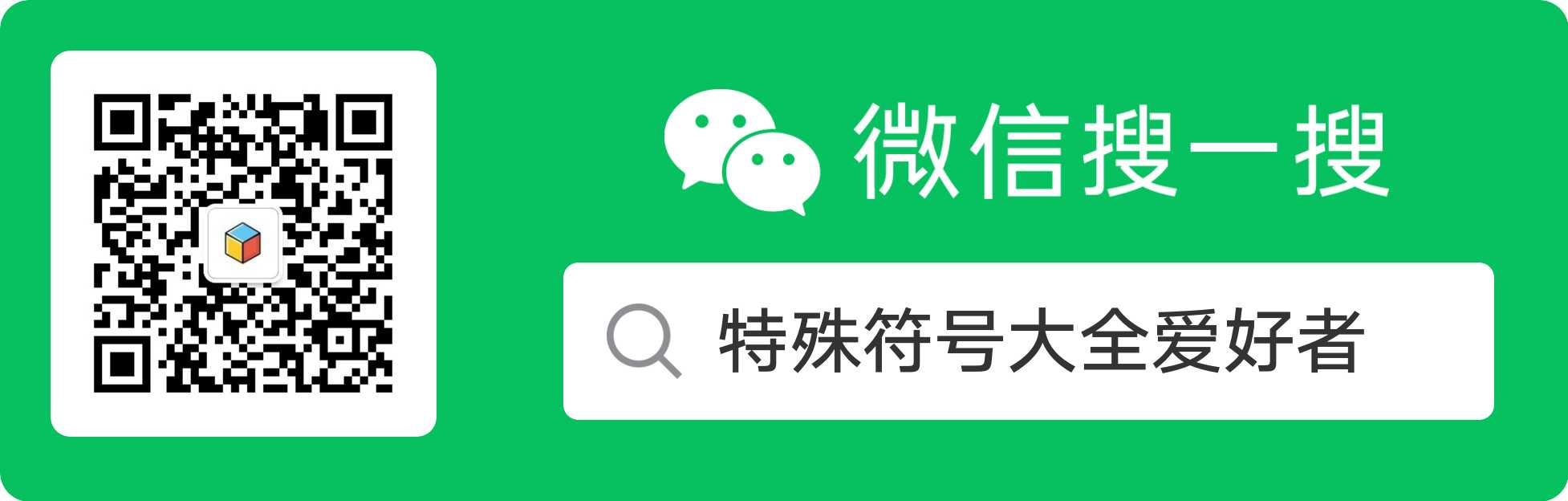# 常用30个ASCII表情和含义

ASCII 表情 含义 ASCII 表情 含义

:-D 开心 :-( 不悦
:-P 吐舌头 :-* 亲吻
;-) 眨眼 :-x 闭嘴
<※ 花束 :-O 惊讶
\$_\$ 见钱眼开 @_@ 困惑
>_< 抓狂 T_T 哭泣
= =b 冒冷汗 >3< 亲亲
≧◇≦ 感动 ＝ ＝＃ 生气
(×_×) 晕倒 (︶︿︶) 不满
(=^_^=) 喵喵 (￣﹁￣) 流口水
(T_T) 哭泣 ╮(￣▽￣)╭ 两手一摊
╭（╯_╰）╭ 路过 (*+﹏+*)~@ 受不了
*\(^_^)/* 为你加油 づ￣ 3￣)づ 飞吻
b（￣▽￣）d 竖起大拇指 (￣(工)￣) 大狗熊
^(oo)^ 猪头 Orz 我服了你